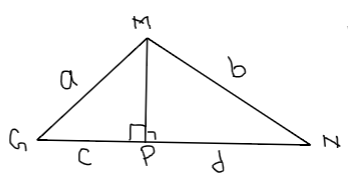Courses
Courses for Kids
Free study material
Free LIVE classes
MoreLIVE
Join Vedantu’s FREE Mastercalss

# In the given figure, $MGN$ is a triangle and $MP$ is perpendicular to $GN$. If $MG = a$ units, $MN = b$ units, $GP = c$ units and $PN = d$ units, prove that $\dfrac{{(a - b)}}{{(c - d)}} = \dfrac{{(c + d)}}{{(a + b)}}.$Verified
364.8k+ views
Hint: Here the given triangle is a right angled triangle, we can use Pythagoras theorem.
In the given figure, $\Delta GMP$ is a right angled triangle. So using Pythagoras theorem, we’ll get:
${(MP)^2} + {c^2} = {a^2}, \\ \Rightarrow {(MP)^2} = {a^2} - {c^2} .....(i) \\$
$\Delta MNP$ is also a right angled triangle. So using Pythagoras theorem again, we’ll get:
${(MP)^2} + {d^2} = {b^2}, \\ \Rightarrow {(MP)^2} = {b^2} - {d^2} .....(ii) \\$
From equation $(i)$ and $(ii)$, we have:
$\Rightarrow {a^2} - {c^2} = {b^2} - {d^2}, \\ \Rightarrow {a^2} - {b^2} = {c^2} - {d^2}, \\ \Rightarrow (a + b)(a - b) = (c + d)(c - d), \\ \Rightarrow \dfrac{{(a - b)}}{{(c - d)}} = \dfrac{{(c + d)}}{{(a + b)}}. \\$
This is the required proof.
Note: In any triangle, perpendicular drawn from a vertex to the opposite side always divides the triangle into two right angled triangles. We can use Pythagoras theorem for both the triangles separately.
Last updated date: 04th Oct 2023
Total views: 364.8k
Views today: 5.64k